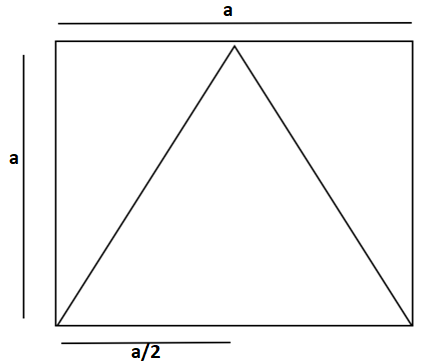Courses
Courses for Kids
Free study material
Offline Centres
MoreLast updated date: 07th Dec 2023
Total views: 383.7k
Views today: 3.83k

# A conical solid block is exactly fitted inside the cubical box of side ‘a’ then the volume of the conical solid block is $\dfrac{{4\pi {a^{3n}}}}{3}$. Is this statement true? Justify your answer.Verified
383.7k+ views
Hint: In this problem we will first find the volume of the solid cone and the volume of the cubical box to check if the given statement is true or false.

Volume of cubical box = ${(side)^3}$ = ${a^3}$.Now, according to the question, if the solid conical block is exactly fitted inside the cubical box, then we can see that the radius (r) = $\dfrac{a}{2}$ and height (h) = a.
So, volume of conical solid block = $\dfrac{{\pi {r^2}h}}{3}$ = $\dfrac{{\pi {{\left( {\dfrac{a}{2}} \right)}^2}a}}{3}$ = $\dfrac{{\pi {a^3}}}{{12}}$.
So, the given statement is not true because, the given volume of conical block is greater than the volume of cubical block in which the conical block is fitted which is not possible. The correct volume of solid conical block fitted inside the box is $\dfrac{{\pi {a^3}}}{{12}}$.